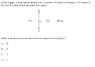# Magnetic field caused by a current-carrying wire

Ipos Manger

In the picture.

## Homework Equations

Right/left hand rule?

## The Attempt at a Solution

I have tried applying the right hand rule to this situation, but they only give me the current and nothing else, so i can't determine the direction of the magnetic field in the point P.

#### Attachments

•Sin título.png
9.2 KB · Views: 524

Homework Helper
Gold Member
Yes, you can determine the direction of the field at P. Can you describe what the magnetic field lines look like around a straight wire that's carrying current? Can you explain the "right hand rule"?

Ipos Manger
Yes, you can determine the direction of the field at P. Can you describe what the magnetic field lines look like around a straight wire that's carrying current? Can you explain the "right hand rule"?
You can use that "thumb-rule" with the right hand? As the current is going into the paper, the magnetic field would be in a clock-wise direction. On the other hand, the lines would be circles in which the magnetic field is in a clock-wise direction.

The "right hand rule" is used because the magnetic force is always normal to the magnetic field?

Homework Helper
Gold Member
Right, the magnetic field lines will circle around the wire in a clockwise direction. Knowing that, can you see what direction the field will be at point P?

Ipos Manger
So i just sort of "expand" the magnetic field lines until it reaches the point P? And the direction would be upwards if i draw a "tangent" to the magnetic field (which is in a curve) at that point.

Homework Helper
Gold Member
Correct! The magnetic field vector at a point is tangent to the magnetic field line that passes through the point.

Tarti
Hi Ipos Manager,

TSny was exactly right with her/his hints. It is really of vital importance for you to understand the right hand rule. Once you understand this rule, you will be able to handle most electrostatic problems since you will have understood Ampere's law.
You can verify the right hand rule and get a feeling for it using some vector calculus and the actual form of the current - it does not change in z-direction (translational symmetry) and neither in angular direction (rotational symmetry). The magnetic field has to obey the same functional dependency, $\mathbf{B}(\mathbf{r}) = \mathbf{B}(\rho)$ where $\rho$ is the distance to the wire, $\rho = \sqrt{x^2+y^2}$ (cylindrical coordinates). Now regarding the curl of the magnetic field in cylindrical coordinates will directly reveal that the magnetic field has only a component in angular $\varphi$ direction (complete calculation here) - a verification of the right hand rule on solid grounds.
Remember however that the angular component of the magnetic field still depends on $\rho$!